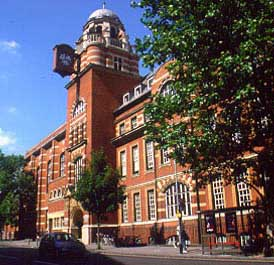# Peter Hydon gives a seminar at City University LondonPeter Hydon is visiting the Department of Mathematics at City University London today (Tuesday 28th October) to give a research seminar on “Difference equations by differential equation methods“.  Around twenty years ago, Peter heard an eminent numerical analyst say, “Any problem involving difference equations is an order of magnitude harder than the corresponding problem for differential equations.” Research since that time has transformed our understanding of difference equations and their solutions. The basic geometric structures that underpin difference equations are now known. From these, it has been possible to develop systematic techniques for finding solutions, first integrals or conservation laws of a given difference equation. These look a little different to their counterparts for differential equations, mainly because the solutions of difference equations are not continuous. However, they are widely applicable and most of them do not require the equation to have special properties such as linearizability or integrability. This talk is an introduction to the most useful of these geometrically-based methods for difference equations.  A link the City University London Mathematics Department is here.